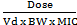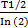# Calculator

## Time above MIC calculator for beta-lactams

 Dose mg Serum half-life, T1/2 Hours Volume of distribution, Vd L/kg Body weight kg Dosing interval Hours Minimal inhibitory concentration, MIC mg/L Time above MIC (T > MIC): %

Formula

%T > MIC = In () xxWhere
ln = natural logorithm;
Vd = volume of distribution (L/kg);
BW = Body weight (kg);
T 1/2 = Serum half-life (hours); and
DI = dosing interval (hours)

Footnote
Calculation according to the one-component first-order elimination model as derived by Turnidge JD 1998